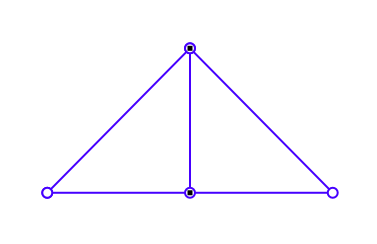# SSAT Elementary Level Math : How to find symmetry of a line

## Example Questions

### Example Question #1 : How To Find Symmetry Of A Line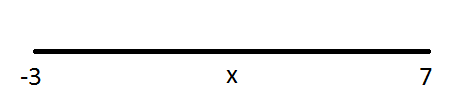is the midpoint of the number line that extends fromto. What is the value of?Explanation:

Find the average of the two endpoints to find their midpoint. To find the average of two numbers, add them together and divide by two.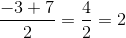### Example Question #1 : Lines

How many lines of symmetry does a square have?Explanation:

A line of symmetry is the imaginary line that you draw through a shape so that you can fold the image over the line and have both halves match exactly. Any regular shape has as many lines of symmetry as it does sides. Since a square has four sides, the correct answer is 4!

### Example Question #2 : Lines

How many lines of symmetry does an octagon have?Explanation:

A line of symmetry divides a shape into two equal and identical halves. Any regular shape has as many lines of symmetry as it does sides. Since octagons have eight sides, the correct answer is 8.

### Example Question #3 : Lines

How many lines of symmetry does a rectangle have?Explanation:

A line of symmetry is the imaginary line that you draw through a shape so that you can fold the image over the line and have both halves match exactly. Any regular shape has as many lines of symmetry as it does total sides. Since a rectangle has four sides, the correct answer is 4!

### Example Question #1 : How To Find Symmetry Of A Line

The length of a certain line isinches. If this line is cut in half, how long is each half?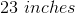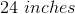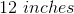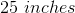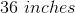Explanation:

Divide the length of the line by 2 to find the length of each half: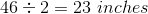### Example Question #2 : How To Find Symmetry Of A Line

How many lines of symmetry does an octagon have?Explanation:

A line of symmetry is the imaginary line that you draw through a shape so that you can fold the image over the line and have both halves match exactly. Any regular shape has as many lines of symmetry as it does sides. Since an octagon has eight sides, the correct answer is.

### Example Question #3 : How To Find Symmetry Of A Line

How many lines of symmetry does the figure have? This is not an equilateral triangle.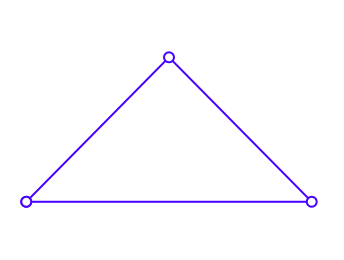There is only one line of symmetry in this figure. It would be right down the middle.